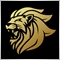# Stop Creating object - HELP98

Hi.

I'm very new to coding so this is probobly done horobly wrong but i still get what i want to happen to happen but to often.

In the code bellow i creat objects at specific creatiria but my problem is that it keeps creating objects with every pip.

How can i make this code just creat one object if the createria is meet?

```//+------------------------------------------------------------------+
//|                                                         test.mq4 |
//|                        Copyright 2015, MetaQuotes Software Corp. |
//|                                             https://www.mql5.com |
//+------------------------------------------------------------------+
#property version   "1.00"
#property strict

int      TimeA=0;
int      TimeB=24;
int      StartHour=TimeA - Hour()*-1;
int      ObjectHour1=StartHour-1;
int      ObjectHour2=StartHour+1;

int      objcount=0;
int      ObjectTime=Time[StartHour];
int      RectangleTime1=Time[ObjectHour1];
int      RectangleTime2=Time[ObjectHour2];

double   SumOfPip=0;
double   pip=1234;
double   HighValue=iHigh(0,PERIOD_H1,StartHour);
double   LowValue=iLow(0,PERIOD_H1,StartHour);

//+------------------------------------------------------------------+
//|                        START Function                            |
//+------------------------------------------------------------------+

int start()
{

if(Hour()>=TimeA && Hour()<TimeB)
{
process();

}
return(0);
}
//+------------------------------------------------------------------+

//+------------------------------------------------------------------+
//|      Entery Point + Ad Intry Objects HLINE                       |
//+------------------------------------------------------------------+

int process(){

double SumOfHighValueLowValue=DoubleToStr(HighValue - LowValue, Digits);

SumOfPip=SumOfHighValueLowValue;
pip=DoubleToStr(Close, Digits);
function_2();

Print(StartHour,"  ",ObjectHour1,"  ",ObjectHour2);

//+------------------------------------------------------------------+
ObjectCreate("line"+objcount,OBJ_RECTANGLE,0,RectangleTime1,HighValue,RectangleTime2,LowValue);

ObjectSet("line"+objcount,OBJPROP_COLOR,Green);
ObjectSet("line"+objcount,OBJPROP_WIDTH,1);

objcount++;
//+------------------------------------------------------------------+
ObjectCreate("line"+objcount,OBJ_HLINE,0,ObjectTime,LowValue,0,0);

ObjectSet("line"+objcount,OBJPROP_COLOR,Red);

objcount++;
//+------------------------------------------------------------------+

return(0);
}
//+------------------------------------------------------------------+
//|   END "Process"                                                  |
//+------------------------------------------------------------------+

//+------------------------------------------------------------------+
//+------------------------------------------------------------------+

int function_2(){

//+------------------------------------------------------------------+

ObjectSet("line"+objcount,OBJPROP_COLOR,Blue);
ObjectSet("line"+objcount,OBJPROP_WIDTH,4);

objcount++;
//+------------------------------------------------------------------+

return(0);
}
//+------------------------------------------------------------------+```

Hope to get some helpful pointers here.

// MathiasModerator
12372

check if the object already exists then skip creation once it is found.

ObjectFind

The function searches for an object having the specified name. There are two variants of the function:

The function searches the object with the specified name:

 ```int  ObjectFind(    string   object_name   // object name    );```

Parameters

chart_id

[in]  Chart identifier.

object_name

[in]  The name of the object to find.

Return Value

If successful the function returns the number of the subwindow (0 means the main window of the chart), in which the object is found. If the object is not found, the function returns a negative number.98

thank you.

That helpt